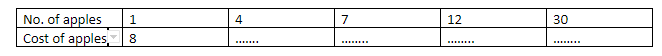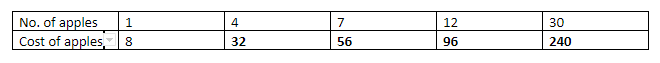QuestionAnswers

# Fill the table.Verified
130.5k+ views
Hint: We need to calculate the value of the cost of apples, from the given table, and we are given that the cost of 1 apple is Rs. 8. So, we will use the unitary method to find the cost of 4,7,12 and 30 apples.

According to the question we need to find the value of 4 apples, 7 apples, 12 apples and 30 apples.
Now, in the question, using unitary method-
Given, Cost of 1 apple is Rs. 8,
Then cost of 4 apples will be $4 \times Rs.8 = Rs.32$
If, cost of 1 apple is Rs. 8
Then, cost of 7 apples will be $7 \times Rs.8 = Rs.56$
If, cost of 1 apple is Rs 8
Then, cost of 12 apples will be $12 \times Rs.8 = Rs.{\text{ }}96$
If, cost of 30 apples will be $30 \times Rs.8 = Rs.240$
Hence the table will now become:Note: Unitary method is not only used to calculate values of more items when the value of one is given. It is also used to calculate the lesser values when value of 1 is given. Also, the use of unitary method or its inverse depends on the situation we are given. For example for calculation costs of things we will use the multiplying or dividing factor, whereas for quantities like work, we will use the inverse unitary method, since 1 person will do lesser work in 1 day as compared to the work done by 20 people in 1 day.